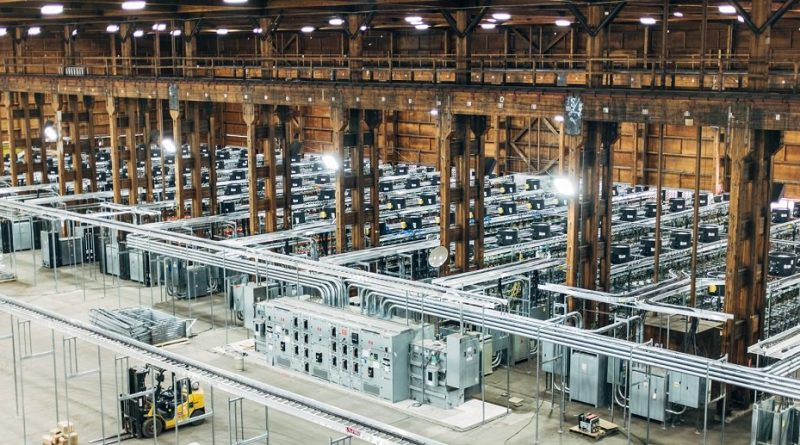# Price of Energy - Q3 2019The exercise as we have been calculating it here is as follows: we calculate the cost of production of coin using energy, and then we calculate the cost of purchasing energy using coin.

Lets start with a quick look at the hash rate and reward so we can say how many satoshis a double SHA256 is worth. We are still in the era of 12.5 BTC coinbase, so that's 12.5*6*24=1800 BTC per day. Tack on 50 or so BTC in fees, call it 1850. That's 0.0214 BTC per second, a whopping 2.14 million satoshi every second.

What else happens in a second? No no, what else related to bitcoin mining happens every second? Well, 90 exahashes are performed by the miners. How many is that? 9.0 * 10^18 double sha256 hashes.

It works out to 2.38*10^-14 satoshis per double hash.

That's not a lot of money. Get cracking!

Next we have a tally of some of the top hardware being advertised:

Bitfury BlockBox: 14PH/S 1.1 MW 79 W/T
Ebang Ebit E12 44TH/S 57 W/T
Canaan Avalon 166-68T 68TH/S 3196W
Bitmain Antminer S17Pro 53TH/S 39 J/TH

Lets just say we are spending something like 50 W of power to keep our boxes spinning at a terahash per second. Surely some people are doing a lot better than what we can dig up in 5 minutes online, but probably some people are doing worse. So what's our global energy hit at 90 EH/s? Well 90 EH is 90 thousand PH which is 90 million TH. That's 4.5 billion Watts. It's a much lower estimate than what some report as the world bitcoin mining energy cost.

If a Watt-second, or Joule, gets us 1/50 of a TH, that's 2*10^10 hashes per Joule. We now have sats per hash, and joules per hash. Our energy cost in sats per joule works out to be about:

C_1 = 0.5 msat / Joule.

That's how much btc we can produce with a Joule of energy, minus overhead.

So our difficulty has gone up a bit, and our hardware has improved a bit, but our price of energy hasn't moved much.

--------

How about the other direction? The range of prices of energy sources in fiat is, well as you'd expect from using fiat currency, literally all over the map: differing by factors of an order or two of magnitude. So lets take an arbitrary cost of \$0.05 USD per kwh, just because this is a number used by Camilo Mora et al. in a Nature Climate Science article, and it's lower than average but that's probably what you want for this application. The price of a dollar today is 1/9350 BTC apparently, so that puts a kwh in at a whopping 535 satoshis per kwh. A kwh is 3.6*10^6 joules, so this gives us:

C_2 = 0.15 msat / Joule

That's how much it costs us to buy a Joule of energy.

The difference between those two bold figures is what drives the expansion of the bitcoin mining industry, and is something likely to remain until something like a free market in energy appears on our lovely little spaceship aka Earth.

The ratio C1/C2 calculated here for BTC is about 4 or 5. Compare that to the ratio for fiat currency: 10^20 or so.

---------------